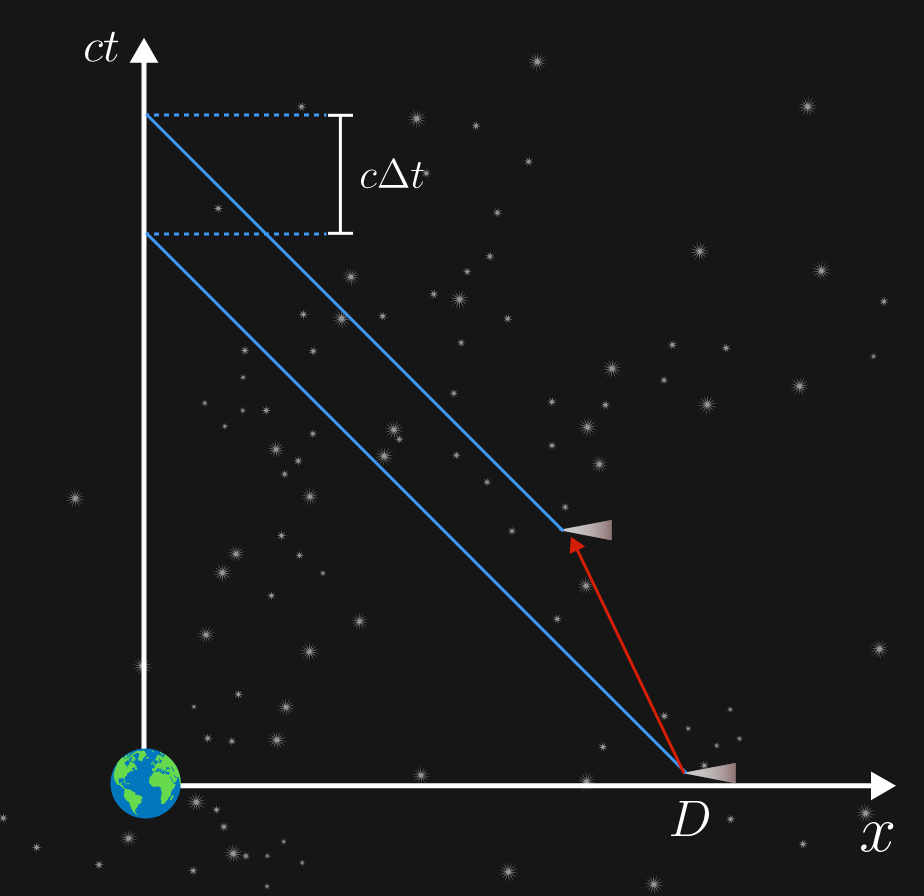# Incoming!A spacecraft approaching Earth very quickly is observed at a distance of $D.$ This has never happened before, so you check your instruments for time $\Delta t,$ and then you check the position of the craft again.

Now you can see it at a distance of $D- 3 c \Delta t,$ where $c$ is the speed of light.

The craft is traveling at a constant speed of $v$ directly toward Earth. What is $v?$

Assume you have made your measurements from an inertial frame very close to Earth.


Hint: The correct solution requires no transformation.

×